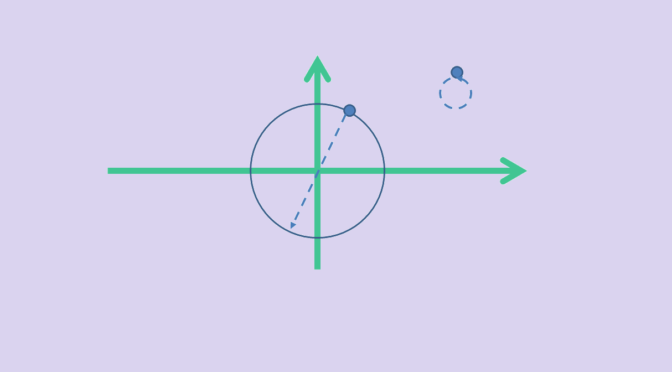# Non linear map preserving Euclidean norm

Let $$V$$ be a real vector space endowed with an Euclidean norm $$\Vert \cdot \Vert$$.

A bijective map $$T : V \to V$$ that preserves inner product $$\langle \cdot, \cdot \rangle$$ is linear. Also, Mazur-Ulam theorem states that an onto map $$T : V \to V$$ which is an isometry ($$\Vert T(x)-T(y) \Vert = \Vert x-y \Vert$$ for all $$x,y \in V$$) and fixes the origin ($$T(0) = 0$$) is linear.

What about an application that preserves the norm ($$\Vert T(x) \Vert = \Vert x \Vert$$ for all $$x \in V$$)? $$T$$ might not be linear as we show with following example:$\begin{array}{l|rcll} T : & V & \longrightarrow & V \\ & x & \longmapsto & x & \text{if } \Vert x \Vert \neq 1\\ & x & \longmapsto & -x & \text{if } \Vert x \Vert = 1\end{array}$

It is clear that $$T$$ preserves the norm. However $$T$$ is not linear as soon as $$V$$ is not the zero vector space. In that case, consider $$x_0$$ such that $$\Vert x_0 \Vert = 1$$. We have:$\begin{cases} T(2 x_0) &= 2 x_0 \text{ as } \Vert 2 x_0 \Vert = 2\\ \text{while}\\ T(x_0) + T(x_0) = -x_0 + (-x_0) &= – 2 x_0 \end{cases}$